# A Beginner's Guide to Linear Regression Models in Python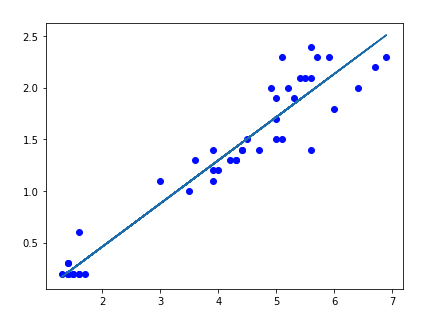Does your team prefer Python over R? Or are you looking to brush up your on your Python skills? We'll walk through a simple example of a linear regression model using the scikit-learn library in Periscope's Python/R Integration. In this exercise, we will also follow guiding principals on creating training and testing datasets.

Here is some information from a fictional gaming company.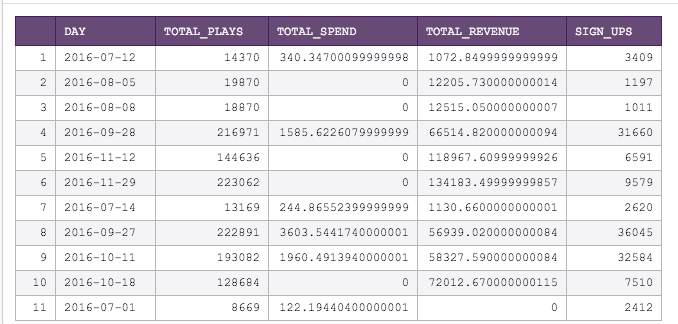That's a lot for a human to parse through. Let's select the "Scatter" chart type in Periscope and click through the different variables to explore possible correlations we can further investigate. Specifically, I want to see if I can predict the revenue for this gaming company based on how many plays have been completed.

Plotting total_plays on the x axis and total_revenue on the y axis, it looks like there's definitely a correlation here worth exploring! Let's dig deeper...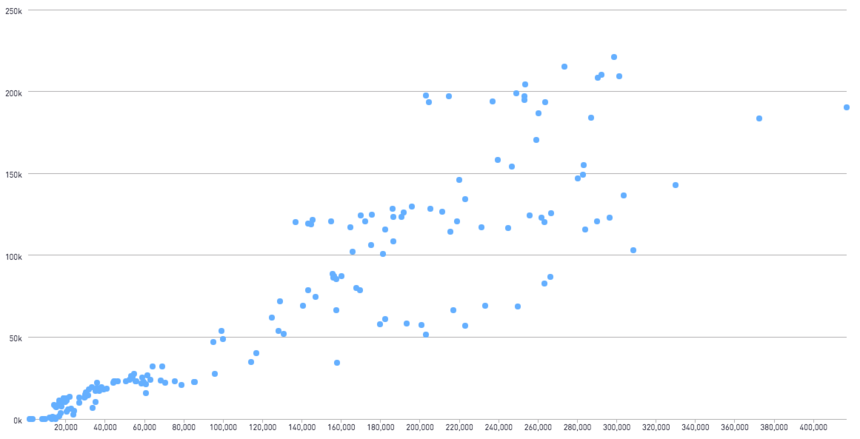There are 2 main steps when creating a model:

1. Building the model on a training data set
2. Testing the model on a testing dataset

This requires us to create 2 subsets of our data. The first subset will be what we use to train our model. Then, we will apply that model onto the second subset. We then can take a look at the difference between the actual values for this subset versus the predicted values.

Let's get started with Python! In the example below, we use Python 3.6.

To begin setting this up, let's first import our libraries, and define what we want our X (our explanatory variable) and Y (response variable) to be.

```import pandas as pd
from sklearn.linear_model import LinearRegression
from sklearn.model_selection import train_test_split

X=df["total_plays"].reshape(-1, 1)
Y=df["total_revenue"].values```

General guidance suggests that we use 70% of our data in our training dataset, and 30% for testing. It's also imperative that this assignment is random. Scikit-learn has a function called train_test_split specifically designed for this (which is why we imported it earlier)!

`X_train, X_test, y_train, y_test = train_test_split(X, Y, test_size=0.3)`

Great, now let's build our model using the training dataset. Python makes this simple with 2 quick lines of code.

```lm = LinearRegression()
model = lm.fit(X_train, y_train)```

Now let's inspect this model further. A linear model will be in the form:

y*=mx+b

where y* is the predicted value of the response variable (total_revenue) and x is the explanatory variable (total_plays). m is the amount of change in the predicted response with every unit change in the explanatory variable. If you plot x and y*, m is commonly referred to as the slope of the line. b is the predicted y* when x=0. On a graph, this would be the y intercept.

Surfacing the m and b of our model is quite simple! We just need to run a quick print statement:

`print(model.coef_, model.intercept_)`

This returns 0.56 for model.coef (which is our slope, m), and -3675 for model.intercept_ (which is our y intercept, b). Note that the y intercept doesn't make much intuitive sense here (this implies we have negative revenue when there are 0 gameplays). It's important to take any model outputs with a grain of salt, and to understand when it makes sense to use the model, versus when another approach would be more practical.

Another key metric when discussing linear regression models is the R^2 value. The closer this value is to 1, the more likely it is that the data is explained by the linear regression model. We call the R^2 value on the test dataset, as shown below.

`print(model.score(X_test,y_test))`

For the example above, this returns 0.81, which is a fairly strong R^2 value.

We can take this further and look at the difference between the predicted y values and the actual y values. This difference is referred to as the residuals. The code below accomplishes this by (1) calculating the predicted values for Y given the values in X_test, (2) converting the X, Y and predicted Y values into a pandas dataframe for easier manipulation and plotting, and (3), subtracting the actual - predicted y values to reach the residual values for each record in the test dataset.

```predictions = lm.predict(X_test)
test=pd.DataFrame({"total_plays":X_test.flatten(), "actual_revenue":y_test.flatten(), "predicted_revenue":predictions.flatten()})
test["residuals"]=test["actual_revenue"]-test["predicted_revenue"]```

Finally, we return the test dataframe back into periscope

`periscope.output(test)`

Let's look at the model, comparing it to our test data.

```#plot the predicted trend line
plt.plot(X_test.flatten(),y_test.flatten(),'bo',X_test.flatten(), predictions.flatten())

periscope.output(plt) ```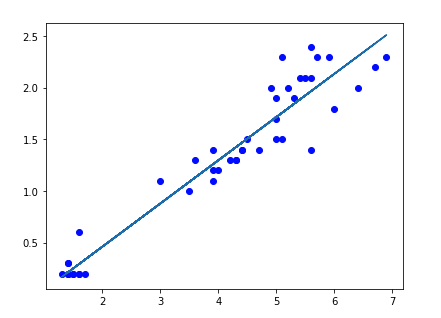We can also plot the residuals by passing in test into periscope.output and using the chart settings below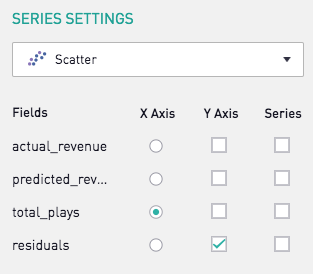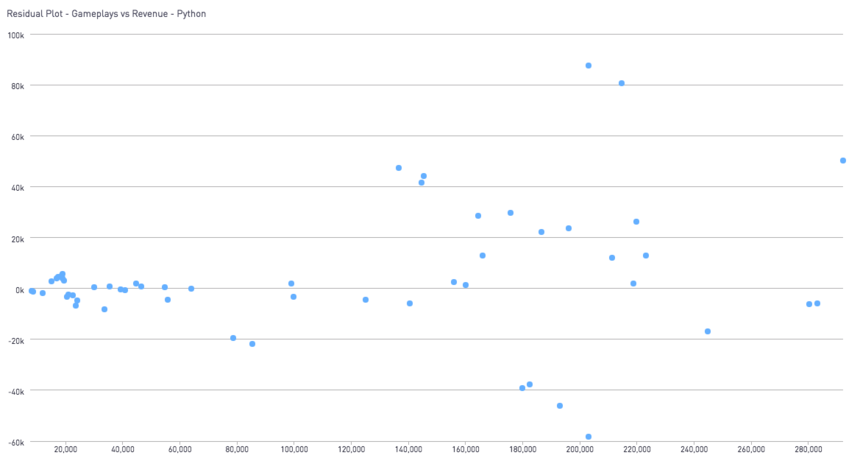In this residual plot, we see that there is no trend in the residuals. This is also further evidence that the data is well explained by a linear model.

New to Python? Did you find this post helpful? Let us know in the comments what you'd like to see!

For more information on linear regression models in Python, I found this blog to be especially helpful!

Prefer R? See the community post here for an R equivalent!

2replies Oldest first
• Oldest first